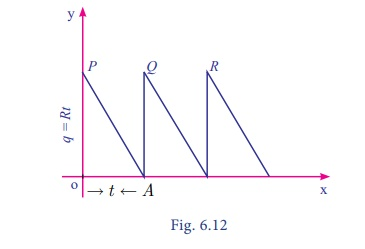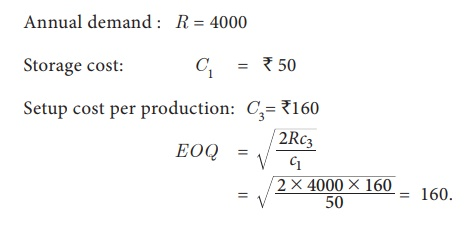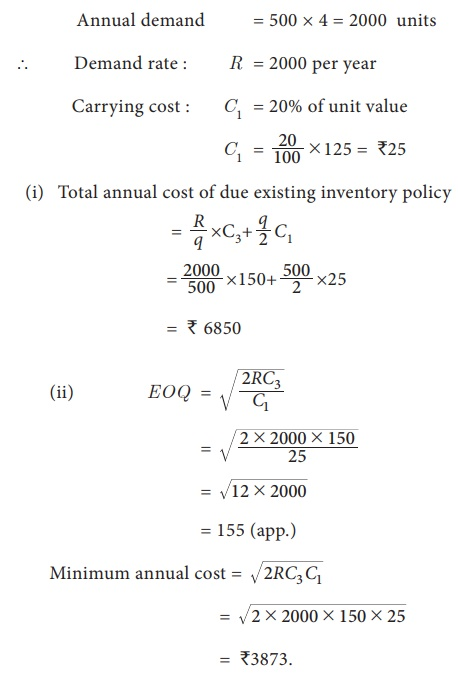Home | | Business Maths 11th std | Economic Order Quantity(EOQ)

# Economic Order Quantity(EOQ)

Economic order quantity (EOQ) is also called Economic lot size formula.

Economic Order Quantity(EOQ):

Economic order quantity is that size of order which minimizes total annual cost of carrying inventory and the cost of ordering under the assumed conditions of certainty with the annual demands known. Economic order quantity (EOQ) is also called Economic lot size formula.

NOTE

The derivation of this formula is given for better understanding and is exempted from examination.

The formula is to determine the optimum quantity ordered (or produced) and the optimum interval between successive orders, if the demand is known and uniform with no shortages.

Let us have the following assumptions.

(i) Let R be the uniform demand per unit time.

(ii) Supply or production of items to the inventory is instantaneous.

(iii) Holding cost is â‚ą C1 per unit time.

(iv) Let there be 'n' orders (cycles) per year, each time 'q' units are ordered (produced).

(v) Let â‚ą C3 be the ordering (set up) cost per order (cycle). Let 't' be the time taken between each order.

Diagrammatic representation of this model is given below:If a production run is made at intervals t, a quantity q = Rt must be produced in each run. Since the stock in small time dt is Rt dt , the stock in period t isExample 6.30

A company uses 48000 units of a raw material costing â‚ą 2.5 per unit. Placing each order costs â‚ą45 and the carrying cost is 10.8 % per year of the average inventory. Find the EOQ, total number of orders per year and time between each order. Also verify that at EOQ carrying cost is equal to ordering cost.

Solution:

Here demand rate R = 48000So at EOQ carrying cost is equal to ordering cost.

Example 6.31

A manufacturer has to supply 12,000 units of a product per year to his customer. The ordering cost (C3) is  â‚ą 100 per order and carrying cost is â‚ą 0.80 per item per month. Assuming there is no shortage cost and the replacement is instantaneous, determine the

(i) economic order quantity

(ii) time between orders

(iii) number of orders per year

Solution:

Demand per year : R = 12,000 units

Ordering cost: C3= â‚ą 100/order

Carrying cost : C1= 0.80/item/month

= 0.80Ă—12 per year

= â‚ą 9.6 per yearExample 6.32

A company has to supply 1000 item per month at a uniform rate and for each time, a production run is started with the cost of â‚ą 200. Cost of holding is â‚ą 20 per item per month. The number of items to be produced per run has to be ascertained. Determine the total of setup cost and average inventory cost if the run size is 500, 600, 700, 800. Find the optimal production run size using EOQ formula.

Solution:

Demand : R = 1000 per month

Setup cost : C3 = â‚ą 200 per order

Carrying cost: C1= â‚ą 20 per item per month.Example 6.33

A manufacturing company has a contract to supply 4000 units of an item per year at uniform rate. The storage cost per unit per year amounts to â‚ą 50 and the set up cost per production run is â‚ą 160. If the production run can be started instantaneously and shortages are not permitted, determine the number of units which should be produced per run to minimize the total cost.

Solution :

Annual demand : R = 4000

Storage cost: C1    =  â‚ą 50To minimize the production cost number of units produced per run is 160 units.

Example 6.34

A company buys in lots of 500 boxes which is a 3 month supply. The cost per box is â‚ą125 and the ordering cost in â‚ą150. The inventory carrying cost is estimated at 20% of unit value.

(i) Determine the total amount cost of existing inventory policy

(ii) How much money could be saved by applying the economic order quantity?

Solution:

Given

Ordering cost per order : C3 = â‚ą150 per order.

Number of units per order: q= 500 units

Annual demand = 500 Ă— 4 = 2000 unitsBy applying the economic order quantity, money saved by a company = 6850â€“3873

= â‚ą2977.

Exercise 6.3

1. The following table gives the annual demand and unit price of 3 itemsOrdering cost is Rs. 5 per order and holding cost is 10% of unit price.

Determine the following:

(i) EOQ in units

(ii) Minimum average cost

(iii) EOQ in rupees

(iv) EOQ in years of supply

(v) Number of orders per year.

2. A dealer has to supply his customer with 400 units of a product per every week. The dealer gets the product from the manufacturer at a cost of â‚ą 50 per unit. The cost of ordering from the manufacturers in â‚ą 75 per order. The cost of holding inventory is 7.5 % per year of the product cost. Find (i) EOQ (ii) Total optimum cost.

Tags : Applications of Differentiation maxima and minima , 11th Business Mathematics and Statistics(EMS) : Chapter 6 : Applications of Differentiation
Study Material, Lecturing Notes, Assignment, Reference, Wiki description explanation, brief detail
11th Business Mathematics and Statistics(EMS) : Chapter 6 : Applications of Differentiation : Economic Order Quantity(EOQ) | Applications of Differentiation maxima and minima